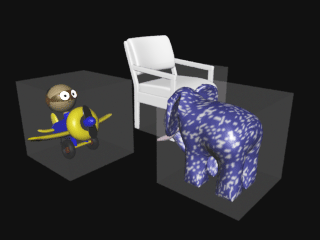### MtorSlim Ribbox Scripting 2

##### Introduction

Slim Ribbox Scripting I provided a couple of simple examples of using TCL expressions in a ribbox. This page provides examples of ribbox scripts that make more extended use of TCL.

All the scripts take this general form. The TCL code must be contained within opening and closing square brackets.

```[
# calculate one or more values using the TCL expr
# procedure ie.
# set foo [expr math_function]
#
# use the TCL return procedure to output one or
# more RIB statements ie.
# return "RIB_Statement \$foo"
]
```
##### Example 1 - setting a surface color

In this example the slim predefined variable "\$pct" is used to set a (grayscale) color based on the sine function. Because the sine function generates values from -1 to 1 the abs() function is used to ensure positive values are assigned to the RIB "Color" statement.

```[
set 2PI 6.282
set cycles 3.0
set comp [expr abs(sin(\$pct * \$2PI * \$cycles))]
return "Color \$comp \$comp \$comp"
]```
##### Example 2 - setting a randomized rotation

In general when we use a function such as rand() what we require is not just random values but repeatable random values. Without performing steps 1 and 2 we would obtain a visually hopeless result because the rotational axis of each cube, on a frame-by-frame basis, would be "dancing" around in an erratic fashion.

figure 1

 To "lock-down" the random number generator in a way that is unique to each cube we use the digits found at the end of the transformation node. For example, the five cubes in the scene had names like, "pCube1", "pCube2" etc. Of course this approach assumes there will always be one or more digits at the end of the name! ```[ # 1 Extract the digit(s) from the end of the # object's transformation node set tokens [split \$OBJPATH |] set node [lindex \$tokens end-1] regsub -all {[^0-9]} \$node "" digits # 2 Lock the rand number generator to the "digits" expr srand(\$digits) # 3 Set an arbitary initial rotation set angle [expr rand() * 90.0] # 4 Make the current rotation equal # to the frame number set angle [expr \$angle + \$F] # 5 Randomize the rotational axis set x [expr rand()] set y [expr rand()] set z [expr rand()] return "Rotate \$angle \$x \$y \$z" ]``` Notes The predefined variable \$OBJPATH contains a string of the following form. `|pCube1|pCubeShape1` By "splitting" on the "|" character we obtain the following TCL list. Note the first item is an empty string. `{} pCube1 pCubeShape1` Therefore, we use the lindex procedure to obtain the next-to-last item ie. index 1. We could index directly using "1" but that would assume our scene graph paths will always consist of two items. The regsub procedure attempts to match against any numerals it finds in the (transformation) node name. For the first cube it will find "1" then "2" for the second cube and so on. figure 2

##### Example 3 - using a custom string attribute to access a prebaked rib

In Maya a custom attribute (aka. channel) can be added to an objects transform node via the Modify->Add Attribute... menu item. In this example, a MEL script adds two string attribute to the shape node of a (selected) object.

```string \$obj[] = `listRelatives -s`;
addAttr -ln bake  -dt "string" \$obj;
addAttr -ln path  -dt "string" \$obj;

setAttr (\$obj + ".bake") -t "string" "teapot.rib";
setAttr (\$obj + ".path") -t "string" "G:/archives";```

The attribute is added to the shape node rather than the transform node so that the TCL script embedded within in the ribbox is able to (more conveniently) access the attribute using slim's built-in "clientcmd" procedure.

```[
set bake [clientcmd "getAttr \$OBJPATH.bake"]
set path [clientcmd "getAttr \$OBJPATH.path"]
if {\$bake != "" && \$path != ""} {
} else {
return "### WARNING: no prebake info!!"
}
]```

The advantage of this approach is that the assignment of a pre-baked rib to a proxy object, say a cuble, can be controlled from the Maya attribute editor. As a consequence a single ribbox, containing the code shown in listing 4, can be attached to several proxy objects. Normally, each proxy object must be assigned its own ribbox that in turn references a specific pre-baked rib.

I am very grateful to Michal Fratczak for suggesting the idea of handling pre-baked RIB's in this way.

This technique would of course require some modification in order to use the more efficient DelayedReadArchive RIB statement.figure 3

##### Example 4 - assigning a TCL expression to a shape node

This example was suggested to me by Michal Fratczak. It involves a cunning way of controlling the behavior of a ribbox by assigning short TCL scripts to the shape node of a proxy object. Suppose two custom attributes are added to the shape node of a poly cube ie.

```string \$shape[] = `listRelatives -s`;
addAttr -ln tcl  -dt "string" \$shape;
addAttr -ln fval -dv 0.2  -k true \$shape;```

Using the "attribute editor" the following text is entered into the "tcl" attribute,

`clientcmd "getAttr \$OBJPATH.fval"`

A ribbox containing the following TCL script is attached to the cube,

```[
set str [clientcmd "getAttr \$OBJPATH.tcl"]
return [eval \$str]
]```

The result of the eval'uation of the short TCL script contained in the "tcl" attribute will be to insert the following text into the output RIB file. In itself this example does not perform any useful task. However, it does demonstrate the fact that Slim's predefined variables (\$OBJPATH for example) can be used in the text of an "external" TCL script that is evaluated somewhat indirectly within the Slim TCL context of a ribbox.

```#slim ribbox RIBBox_5
0.2 <--- result of "indirect" evaluation```